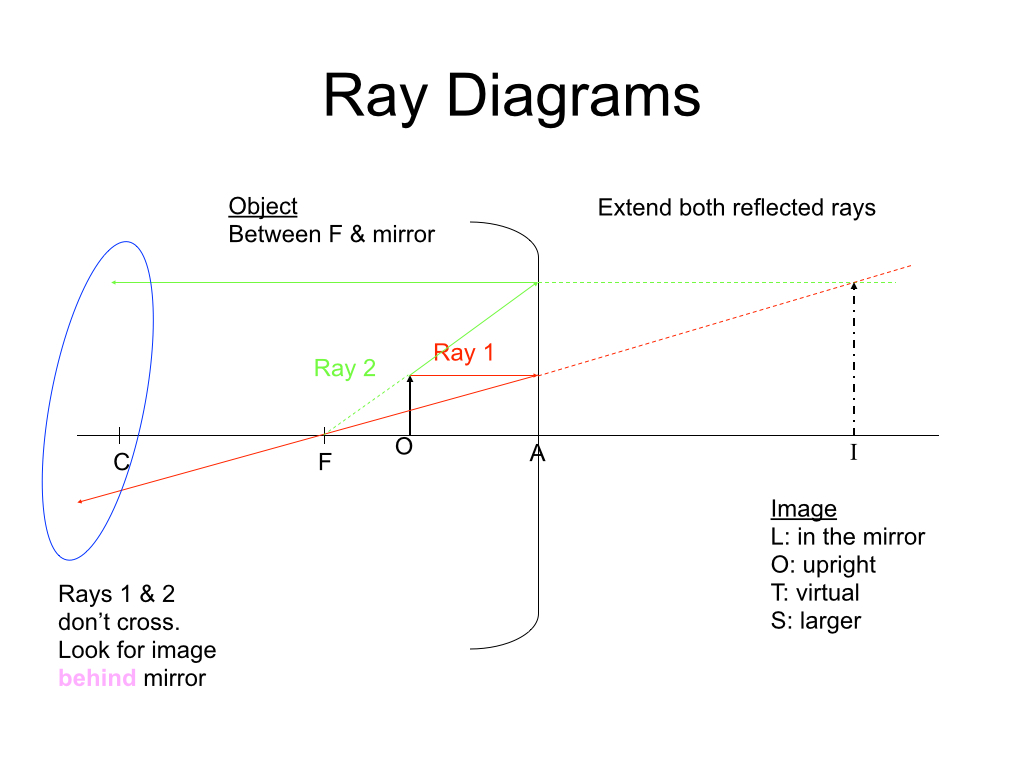# Day 114: Ray Diagrams for Spherical Mirrors

Today, we started by comparing observations from the mirror lab and looking for patterns. After summarizing the properties of images formed by spherical mirrors, we worked on constructing ray diagrams for concave and convex mirrors. We drew three different cases step-by-step together as a class.After drawing ray diagrams, I presented the mirror equation. Students now have three experiences with geometric optics – the lab, ray diagrams, and the mirror equation.

##optics